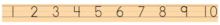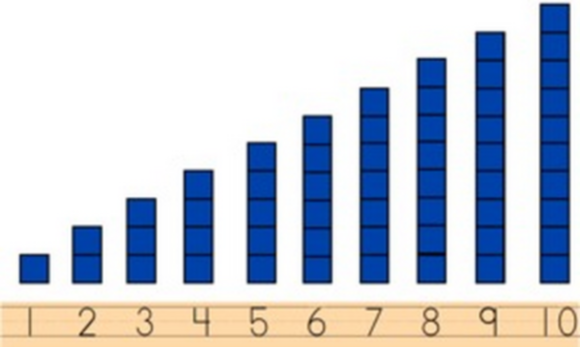• 09/08/13   |   Adjusted: 01/10/17   |   1 file# Number Rods

Author: Illustrative Mathematics

• Description
• Files

Mathematically:

• Addresses standards:  K.CC.B, K.NBT.A.1, and MP.2
• Connects counting, cardinality, and linear ordering of numbers (K.CC.B)
• Builds meaning for numbers 11-19 as ten ones and some more ones (K.NBT.A.1)
• Relates concrete quantities and abstract symbols (MP.2)

In the classroom:

• Uses concrete representation to make the mathematics explicit
• Allows for group or individual work
• Can lead into related discussions (such as one more/one less)

• Making the Shifts

How does this task exemplify the instructional Shifts required by CCSSM?

 Focus Belongs to the major work of kindergarten Coherence Prepares for first grade work in multi-digit operations Rigor Conceptual Understanding: primary in this task Procedural Skill and Fluency: secondary in this task Application: not targeted in this task

Materials:• Unifix cubes or snap cubes, composed into rods with 1-10 cubes (or any counting sequence the class is currently working on within 20).
• If the chosen number range includes numbers greater than 10, then make the rods using two colors so that the set of ten is easily identified (see the image in the solution).• The number line for the chosen number range.

Action:

The students count the number of unifix cubes, then match the rod up to the correct number on the number line. Students can work in pairs or on their own.

• Illustrative Mathematics Commentary and Solution

Commentary

This activity gives students practice counting for meaning. This task also allows students to see the size of the rod grow as the number gets larger.

One variation of this game is to give students a tub of unifix cubes and have them build the rods. However, this version is for students who are proficient counters. Students whose counting skills are still emerging will do better with the number rods already made for them.

As an extension, students can be asked to figure out why 2 colors were used to represent the numbers larger than 10. Also students can be asked what they notice about the cubes as you go from one number to the next (up or down). This could lead to a good discussion on one more and one less.

Materials Note: Very long rods of unifix cubes can break easily. Check to make sure each rod is intact before students start the game. Also instruct students to handle the rods carefully if the rods are longer than ten cubes. If the teacher does not have access to unifix or snap cubes, colored number strips could be made on heavy-duty paper.

Solution:

Students will match the rods to the number line so that each rod is above its number.